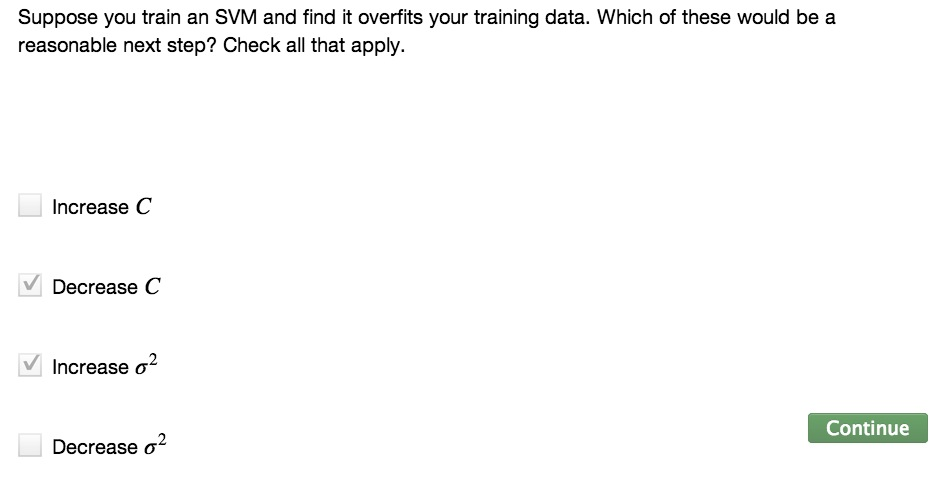• Kernels video to define new features
• This video discussed about what missing in kernels and bias/variance trade off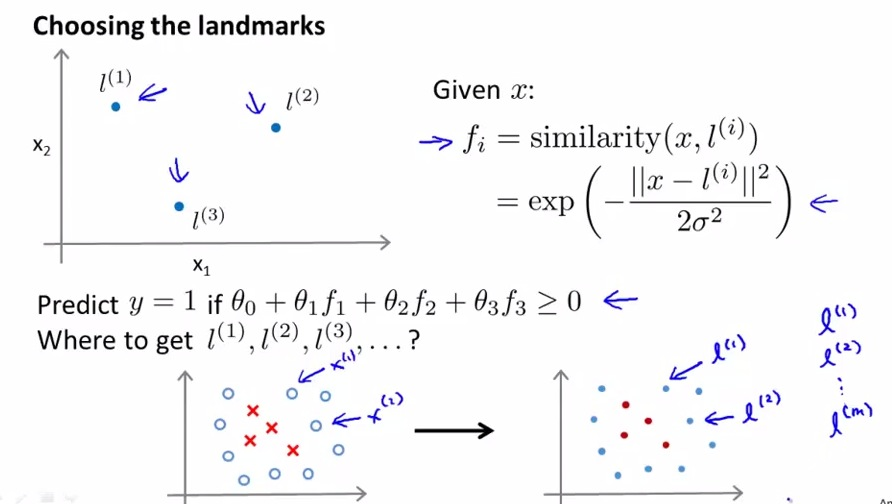• for every training example that we have, put landmark on same location.
• That way we know similarity between landmarks and training example. So we're going to have landmarks as much as training examples.
• This is based on training example on features(let's say x1/x2). The color of graph shown on the right is not matter much.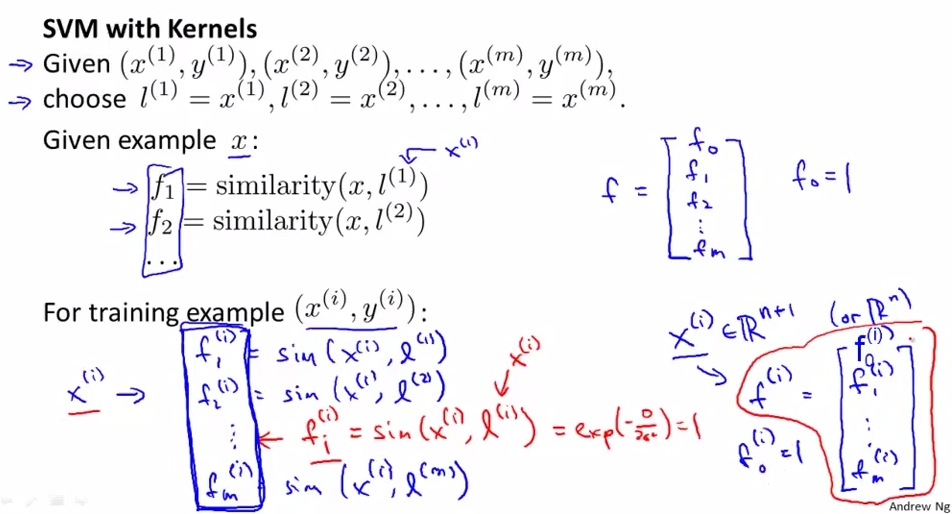• This section is where we putting together SVM with kernels.
• So first of all we're going to make landmarks with m-unit, and assign each landmark with training example x.
• Then for every training example x (can be from training/cross-validation/test set) compute f vector
• f vector is where we compute between all similarity (from f1 to fm) and x, gather all the similarity value to x and wrap it into a vector.
• In other words, by example x(i) would have fi m-dimensional vector, with elements compute from f1 to fm.
• Among all f value, there's should be fi which exactly similar to xi.
• And then, instead of including xi, we're going to include fi, where fi is f m-dimensional vector that each elements calculate similarity to xi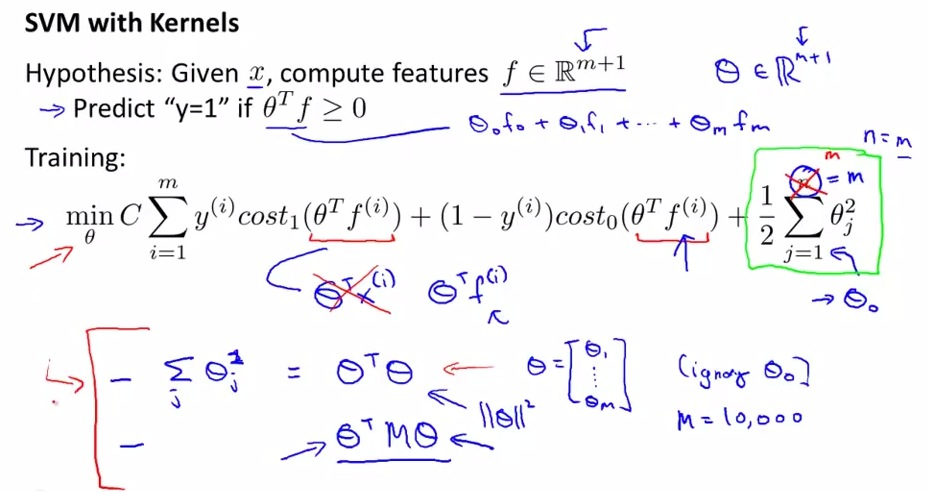• Instead of usual hypothesis that include xi, we're going to replace it with fi.
• So the prediction then change that compute theta transpose multiply with f.
• As we look into the formula each theta will be multiplied by f.
• As f unit is match to m training examples, then the number of theta should be in equal number of training examples
• n = number of features, eventually equals to m (same number of units as training examples).
• How to get parameters (theta) value? We're doing this with SVM training cost function that discussed earlier, in goal is to minimize theta.
• As we can see in the SVM formula, we already replace xi with fi.
• Now, the regularization term is slightly different. We already discussed about n being the same number of m, keep in mind that theta0 is still unregularized.
• For SVM problem, computing f-m is computationally expensive, we're basically have parameters that have m unit.

• So we're going to slightly modify regularization term to be coherent with SVM and make it efficient calculation.
• Instead of having usual regularization term, we're going to multiply thetaT * theta, exclude theta0
• "M" is rescaled version, from kernel SVM, that try to minimize the value of theta
• M is mathematical detail, reason for mathematical efficiency
• Kernels, can be applied to logistic regression using kernels, but can be computationally expensive and can be really slow
• SVM with its character can be going well with kernel, and with other advanced optimization techniques specifically for SVM can be really efficient
• Use external packages, don't write own code, because has tested to be compute really fast. SVM has built-in in common library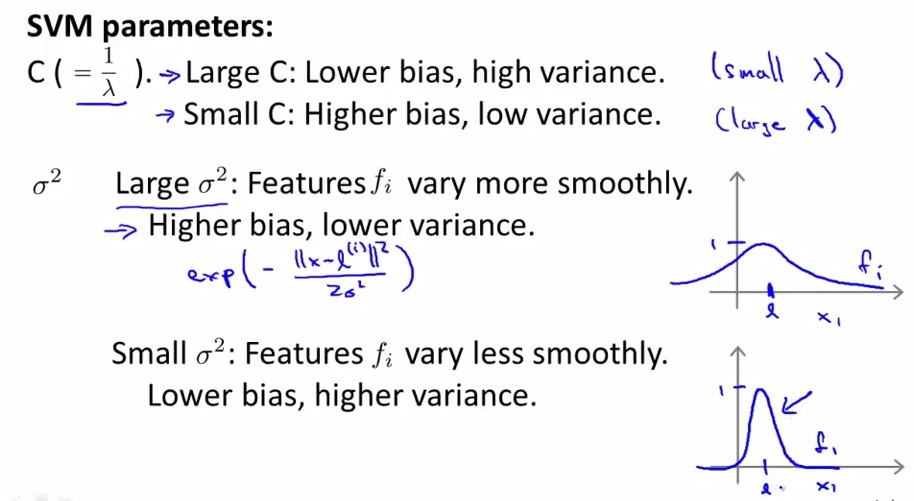• This is a topic of worth mentioning in SVM kernel.
• Choose wisely of C value, as we learn the higher the C value, more overfit, where lower C value, more underfit.
• Then we also know sigma squared of the Gaussian kernel, where fi tends to vary smoothly, long range because bigger sigma, and rapid fall, narrower with smaller sigma.
• In summary these are all SVM in kernels algorithm, and how the behaviour